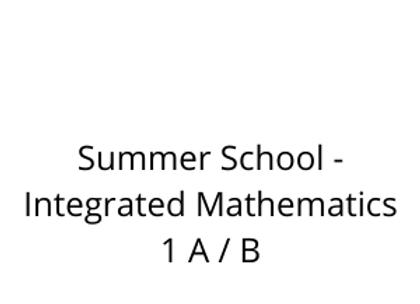# Summer School - Integrated Mathematics 1 A / B

\$275.00Price
`									Integrated Math is a two-semester comprehensive collection of mathematical concepts designed to give you a deeper understanding of the world around you. It includes ideas from algebra, geometry, probability and statistics, and trigonometry, and teaches them as interrelated disciplines. It’s likely that you’ve been studying some form of integrated math since elementary school. In Integrated Math 1A, you will begin with algebra. You will build on your understanding of single-variable and two-variable expressions, equations, and inequalities. You will also learn how to write equations and inequalities to represent and solve word problems.  In Integrated Math 1B, you will explore the connections between algebra and			geometry. You will learn about functions and use them to solve real-world math problems. You will study data collection methods and use different types of data plots to represent and analyze statistical data.			You will learn geometric theorems and rules and write proofs to support them. You will also explore			congruency and similarity of triangles.						`